# Outer contact of circles

Construct a circle k1 (S1; 1.5 cm), k2 (S2; 2 cm), and K3 (S3; 2.5 cm) so that they are always two outer contacts. Calculate the perimeter of the triangle S1S2S3.

o =  12 cm

### Step-by-step explanation: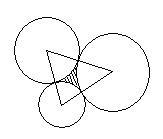Did you find an error or inaccuracy? Feel free to write us. Thank you!Tips to related online calculators

#### You need to know the following knowledge to solve this word math problem:

We encourage you to watch this tutorial video on this math problem:

## Related math problems and questions:

• Outside tangents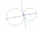Calculate the length of the line segment S1S2 if the circles k1 (S1, 8cm) and k2 (S2,4cm) touch the outside.
• Tangents constructThe circle k is given k (S; 2.5 cm) and an outer line p. Construct a tangent t of the circle that has with a line p angle 60°. How many solutions have the task?
• Construct rhombusConstruct rhombus ABCD if given diagonal length | AC | = 8cm, inscribed circle radius r = 1.5cm
• ConstructConstruct a triangle ABC inscribed circle has a radius r = 2 cm, the angle alpha = 50 degrees = 8 cm. Make a sketch, analysis, construction and description.
• Construction of trapezoidConstruct a trapezoid if b = 4cm, c = 7cm, d = 4,5cm, v = 3 cm (Procedure, discussion, sketch, analysis, construction)
• Circle tangentIt is given to a circle with the center S and radius 3.5 cm. Distance from the center to line p is 6 cm. Construct a circle tangent n which is perpendicular to the line p.
• Complete constructionConstruct triangle ABC if hypotenuse c = 7 cm and angle ABC = 30 degrees. / Use Thales' theorem - circle /. Measure and write down the length of legs.
• Triangle SSAConstruct a triangle ABC if |AB| = 5cm va = 3cm, CAB = 50 °. It is to create the analysis and construction steps.
• Two heights and a side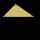Construct triangle ABC when the given side is c = 7 cm, height to side a va = 5 cm and height to side b: vb = 4 cm.
• Construct 1Construct a triangle ABC, a = 7 cm, b = 9 cm with right angle at C, construct the axis of all three sides. Measure the length of side c (and write).
• Draw a trapezoidDraw a trapezoid if given a = 7 cm, b = 4 cm, c = 3.5 cm, diagonal AC = 5cm. Solve as a construction task.
• Construct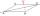Construct a rhombus ABCD, if the size of the diagonal AC is 6 cm and diagonal BD 8 cm long.
• Trapezoid - constructionConstruct a trapezoid KLMN, where: k = 9 cm, l = 4 cm, m = 5 cm and angle α = 45°
• Sides od triangleSides of the triangle ABC has length 4 cm, 5 cm and 7 cm. Construct triangle A'B'C' that are similar to triangle ABC which has a circumference of 12 cm.
• Construction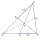Construction the triangle ABC, if you know: the size of the side AC is 6 cm, the size of the angle ACB is 60° and the distance of the center of gravity T from the vertex A is 4 cm. (Sketch, analysis, notation of construction, construction)
• Rhombus EFGHConstruct the rhombus EFGH where e = 6.7cm, height to side h: vh = 5cm
• Right triangleDraw a right triangle ABC if |AB| = 5 cm |BC| = 3 cm, |AC| = 4 cm. Draw Thales circle above the hypotenuse of the triangle ABC.